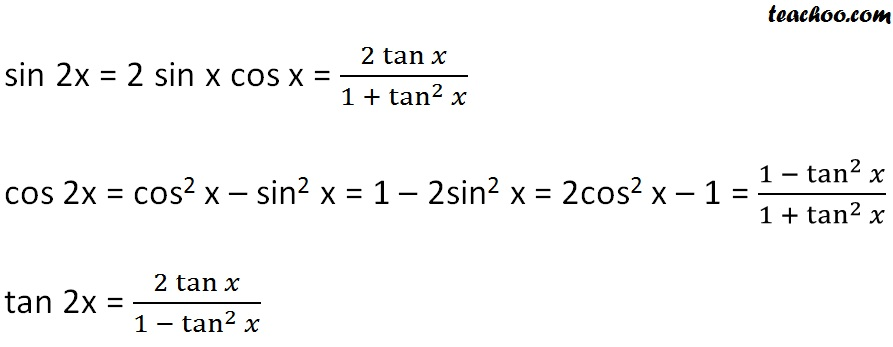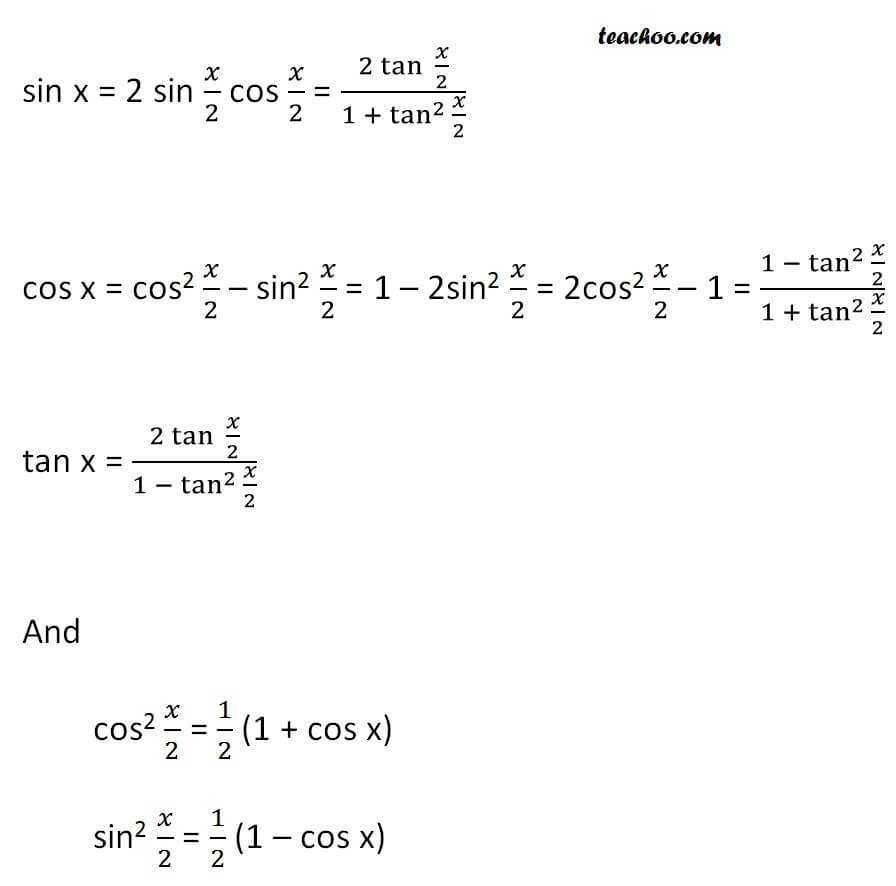2x 3x formula - Proving

Chapter 3 Class 11 Trigonometric Functions
Concept wise

We know from double angle formula thatThese identities can also be used to reduce anglesGet live Maths 1-on-1 Classs - Class 6 to 12

### Transcript

We know from double angle formula that sin 2x = 2 sin x cos x = 2 tan x / (1 + tan^2 x) cos 2x = cos^2 x - sin^2 x = 1 - 2 sin^2 x = 2 cos^2 x - 1 = 1 - tan^2 x / 1 + tan^2 x tan 2x = 2 tan x / (1 - tan^2 x) These identities can also be used to reduce angles sin x = 2 sin x/2 cos x/2 = 2 tan x/2 / (1 + tan^2 x/2) cos x = cos^2 x/2 - sin^2 x/2 = 1 - 2 sin^2 x/2 = 2 cos^2 x/2 - 1 = 1 - tan^2 x/2 / 1 + tan^2 x/2 tan x = 2 tan x/2 / (1 - tan^2 x/2)